### Home > MC2 > Chapter 2 > Lesson 2.2.4 > Problem2-77

2-77.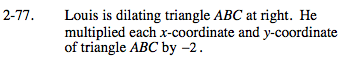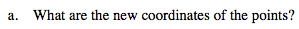Use the graph to find the coordinates of Points A, B, and C. Then multiply each value by −2. For example, Point A is originally (−3,2). It's new coordinate will be (6,−4). Find Points B and C.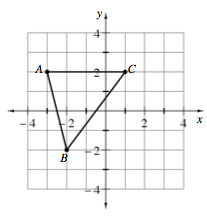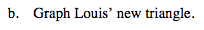After multiplying each x-coordinate and y-coordinate by −2, plot the new points.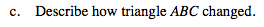Its sides are twice as long and rotated 180 degrees.

Use the eTool below to graph Louis' new triangle.
Click the link at right for the full version of the eTool: MC2 2-77 HW eTool